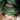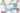Open Source
May 09, 2016

# Neural Networks in JavascriptTine Wiederer
@errorroutine
Neural networks provide the possibility to solve complicated non linear problems. They can be used in various areas such as signal classification, forecasting timeseries and pattern recognition. A neural network is a model inspired by the human brain and consists of multiple connected neurons. The network consists of a layer of input neurons (where the information goes in), a layer of output neurons (where the result can be taken from) and a number of so called hidden layers in between:
For getting a deeper understanding, I recommend checking out Neural Networks and Deep Learning.
Within the last years, multiple Javascript frameworks were developed that can help you to create, train and use Neural Networks for different purposes. In this blog post, you will learn how to set up a Network and use it for classifying images.
A common example for getting started with Neural Networks is the classification of handwritten digits. To achieve good results, a network has to be trained properly. Therefore, we need a set of data called a training set. In our example, we will use the MNIST numbers, a set of thousands of 28x28px binary images of handwritten numbers from 0 to 9:
The MNIST database containing 60,000 examples for training and 10,000 examples for testing can be downloaded from LeCun's website. Instead of downloading the database and converting the data to actual images, we can use the helpful library MNIST digits, which creates test- and training sets automatically.
`const mnist = require('mnist');const set = mnist.set(700, 20);const trainingSet = set.training;const testSet = set.test;`
The above code creates a training set of 700 images and a testset with 20 elements. When creating the sets manually, it is important to make sure there are no duplicate elements in the sets. If you're using the MNIST digits library, this is checked automatically.
After creating the data for training and testing, we can set up the network. We will use the library synaptic.js, which gives us the possibility to create a neural network and configure various parameters. First of all, we have to determine how many input and output neurons are needed. As the size of each image is 28x28px, the number of pixels the network has to take as input is 28 x 28 = 784. The digits should be assigned to one of ten classes, so the number of output neurons will be 10. Furthermore, the network should have at least one hidden layer, which in this example is set to consist of 100 neurons.
The following code sets up the network decsribed above:
`const synaptic = require('synaptic');const Layer = synaptic.Layer;const Network = synaptic.Network;const Trainer = synaptic.Trainer;const inputLayer = new Layer(784);const hiddenLayer = new Layer(100);const outputLayer = new Layer(10);inputLayer.project(hiddenLayer);hiddenLayer.project(outputLayer);const myNetwork = new Network({  input: inputLayer,  hidden: [hiddenLayer],  output: outputLayer,});`
To train the network with our training set, we can use the Trainer provided by synaptic.js. The train() function takes the data used for the training and a list of parameters for the configuration of the trainer.
`const trainer = new Trainer(myNetwork);trainer.train(trainingSet, {  rate: 0.2,  iterations: 20,  error: 0.1,  shuffle: true,  log: 1,  cost: Trainer.cost.CROSS_ENTROPY,});`
As options, you can set the 'rate', which is the learning rate for the training. The 'iterations' define, after how many iterations the training determines. The 'error' is the minimum error that can be reached during the training, if it is achieved, the training stops. By setting the 'shuffle' option, you can specify if the training set is ordered randomly or not. You can find more detailed information about all possible options in the synaptic.js documentation.Colors Of Europe
Interactive Data Visualization (Zeit Online)
Are you interested in a collaboration?
We are specialized in creating custom data visualizations and web-based tools.
`console.log(myNetwork.activate(testSet.input));console.log(testSet.output);`